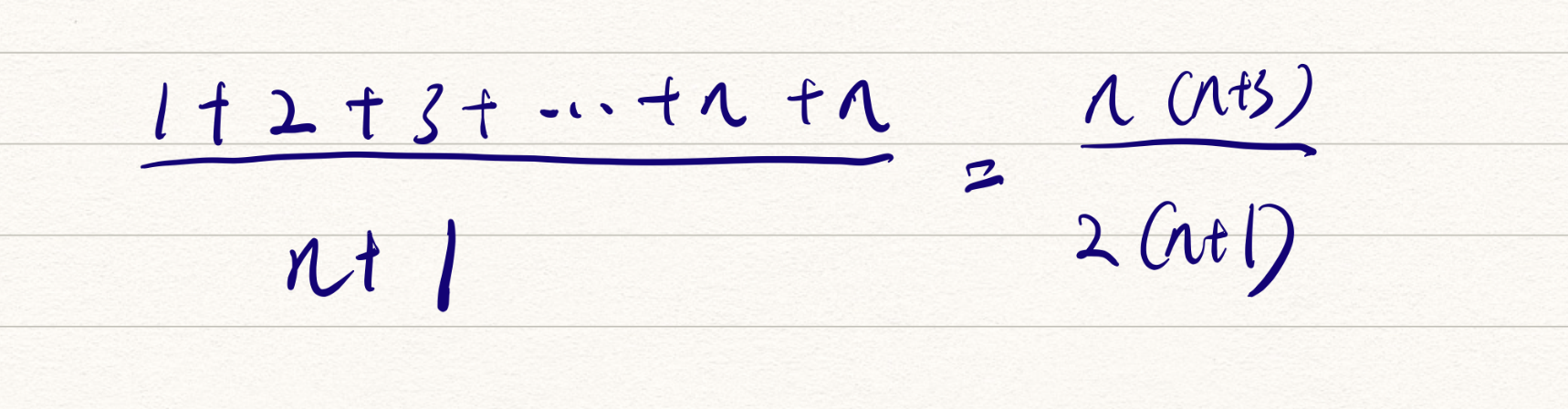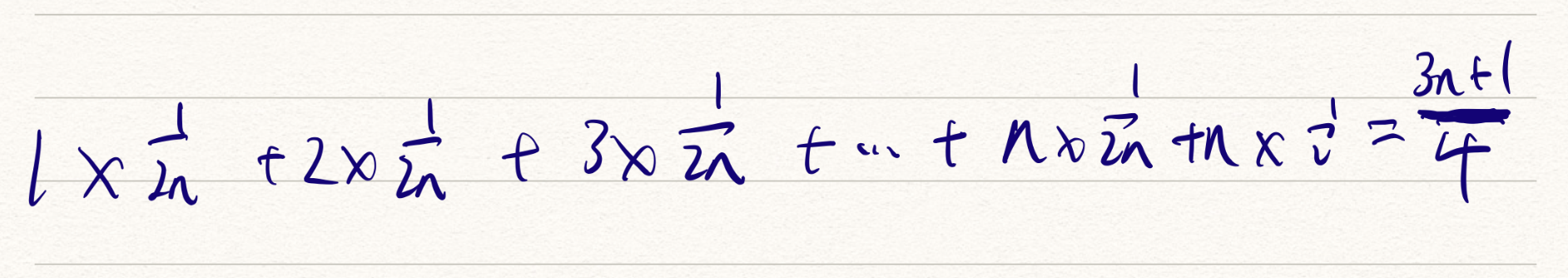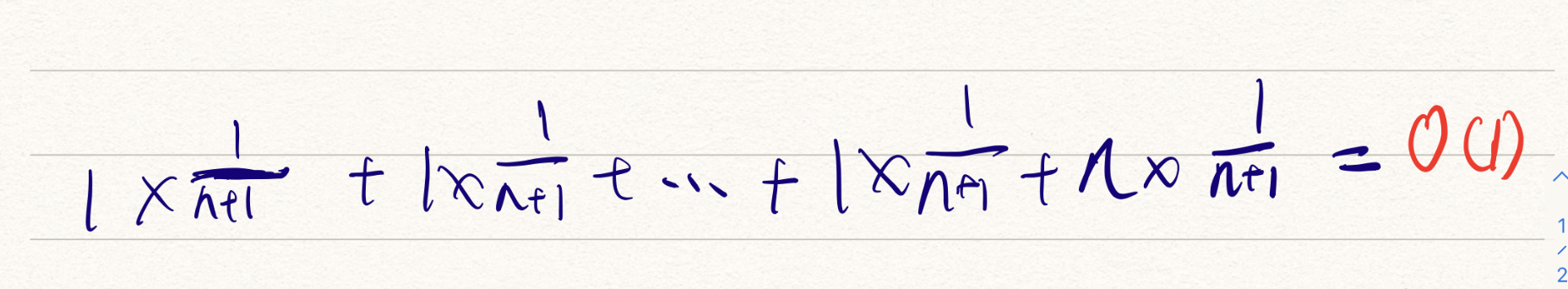• 最好情况时间复杂度 (best case time complexity)
• 最坏情况时间复杂度 (worst case time complexity)
• 平均情况时间复杂度 (average case time complexity)
• 均摊时间复杂度 (amortized time complexity)

### 最好、最坏情况时间复杂度

		/**
* 分析：数据规模是不确定的所以不能忽略，而是要相加 首先要循环 n 次，所以 O(n)
* 另外循环进行判断 pos == x 最好情况是第一次就找到，最坏是最后找到，也就是又循环了 n 次
* O(1) ~ O(n) ，按最坏来 O(n+n) O(2n) 忽略常数 O(n)
*/
int find(int[] array, int n, int x) {
int i = 0;
int pos = -1;
for (; i < n; ++i) {
if (array[i] == x) {
pos = i;
}
}
return pos;
}


【这里我的结论虽然没错，但是过程有问题，问题出在 if 判断上，我好像想复杂了。】

int find(int[] array, int n, int x) {
int i = 0;
int pos = -1;
for (; i < n; ++i) {
if (array[i] == x) {
pos = i;
// 增加了一行找到之后则中断循环的代码
break;
}
}
return pos;
}


【我感觉要引出最好最坏时间复杂度了。】

• 最好情况时间复杂度：最理想情况下执行代码的时间复杂度，对应上面的查找元素位于数组第一个的 O(1)
• 最坏情况时间复杂度：最糟糕情况下执行代码的复杂度，对应上面的遍历整个数组的 O(n)
• 平均情况时间复杂度

### 平均情况时间复杂度

• 平均情况时间复杂度。【这里的关键点：

• 同一代码
• 不同情况
• 时间复杂度有量级差距（个人认为最重要）

### 均摊时间复杂度

// array 代表一个长度为 n 的数组， 方法中的 array.lenght 就是数据规模 n
int[] array = new int[n];
int count = 0;

void insert(int val) {
if (count == array.length) {
int sum = 0;
for (int i = 0; i < array.length; ++i) {
sum = sum + array[i];
}
array = sum;
count = 1;
}
array[count] = val;
++count;
}


• 最理想情况：数组中有空闲空间，只需要将数据插入到下标为 count 的位置即可，此时的时间复杂度是常量 O(1)

• 最坏情况：数组已满，需要遍历求和之后再插入数据，复杂度为 O(n)

• 平均复杂度O(1) ，还是用之前的概率论方法来分析。

• 假设数组长度为 n，根据插入位置不同，可以分为 n 种情况，每种情况的时间复杂度是 O(1)。
• 还有一种额外情况，在数组没有空闲空间时插入一个数组，这个时间复杂度是 O(n)
• 这 n + 1 种情况发生的概率相同 --> 1 / (n+1)
• 根据平均加权求得：最终平均复杂度：O(1)• find 函数在极端情况下，复杂度才是O(1)，但是 insert() 在大部分情况下 复杂度都是 O(1)
• insert O(1) 时间复杂度的插入 和 O(n) 时间复杂度的插入出现的频率很有规律，且存在前后时序关系，一般是 1O(n) 插入之后紧跟着 n-1O(1)循环往复

• 连续操作存在规律
• 大部分情况时间复杂度都很低，个别情况比较高
• 操作之间存在连贯时序关系

Q.E.D.